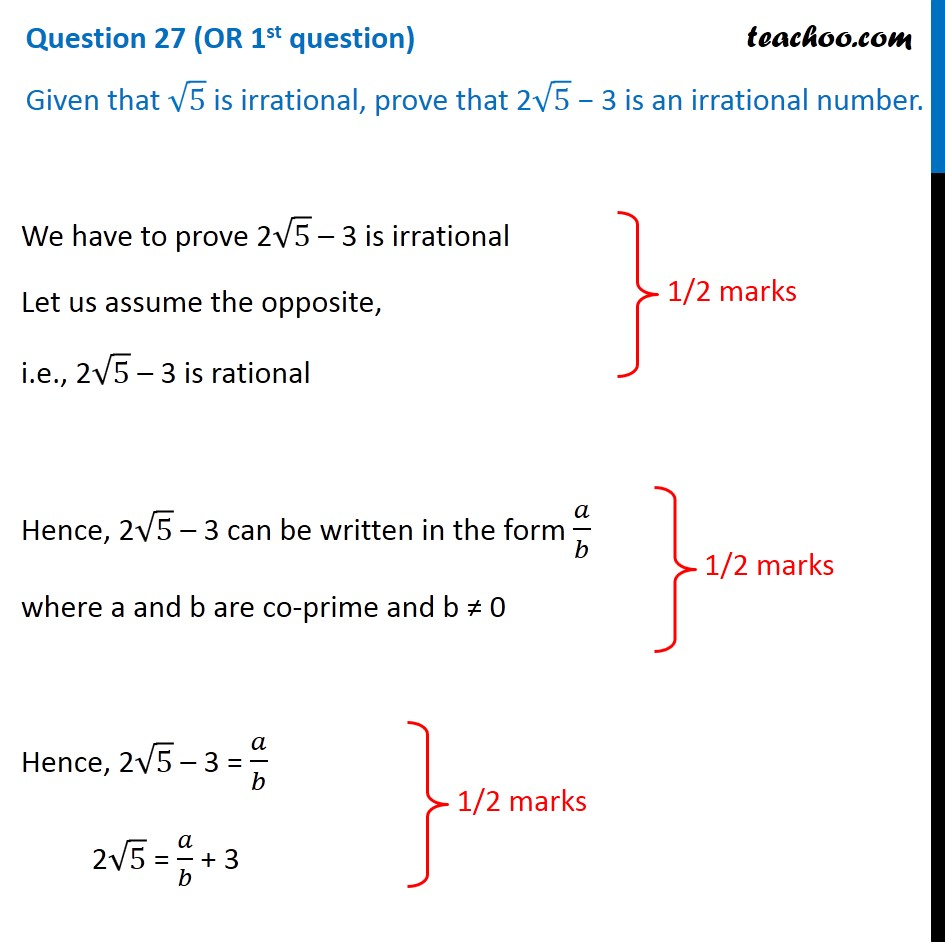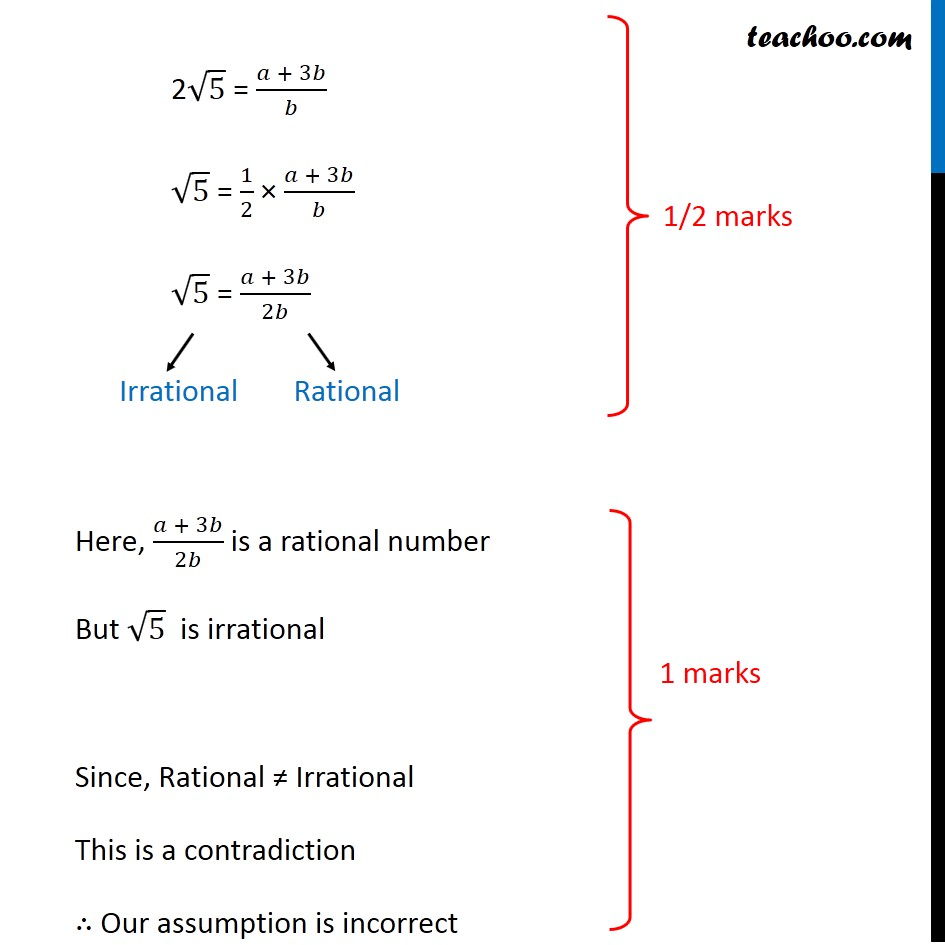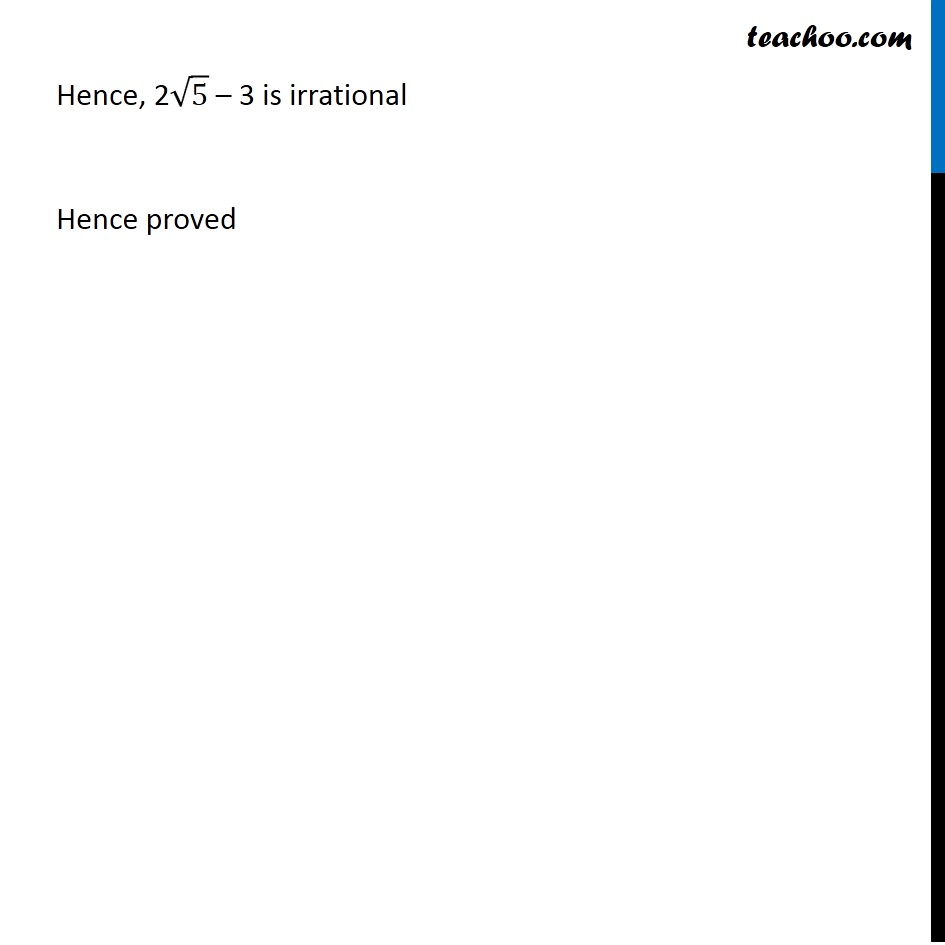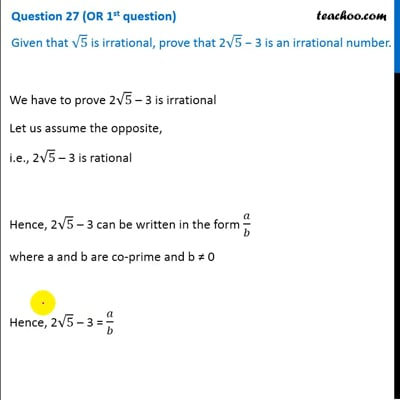CBSE Class 10 Sample Paper for 2020 Boards - Maths Standard

Class 10
Solutions of Sample Papers for Class 10 Boards

## Given that √5 is irrational, prove that 2√5 − 3 is an irrational number.This video is only available for Teachoo black users

Note : This is similar to Ex 1.3, 2 of NCERT – Chapter 1 Class 10

Get live Maths 1-on-1 Classs - Class 6 to 12

### Transcript

Question 27 (OR 1st question) Given that √5 is irrational, prove that 2√5 − 3 is an irrational number. We have to prove 2√5 – 3 is irrational Let us assume the opposite, i.e., 2√5 – 3 is rational Hence, 2√5 – 3 can be written in the form 𝑎/𝑏 where a and b are co-prime and b ≠ 0 Hence, 2√5 – 3 = 𝑎/𝑏 2√5 = 𝑎/𝑏 + 3 2√5 = (𝑎 + 3𝑏)/𝑏 √5 = 1/2 × (𝑎 + 3𝑏)/𝑏 √5 = (𝑎 + 3𝑏)/2𝑏 Here, (𝑎 + 3𝑏)/2𝑏 is a rational number But √5 is irrational Since, Rational ≠ Irrational This is a contradiction ∴ Our assumption is incorrect Hence, 2√5 – 3 is irrational Hence proved